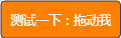# Python变量学习

### 1.变量介绍

``````Teacher="zhangzexiang"
print (Teacher)``````

### 2.用变量进行计算

2.1 这个就是`第1章 打印输出 第2节 引号规则`里面说的给变量名赋值。

``````a=6
b=4
print(a+b)``````

2.2 我们也可以将a+b的计算结果用c来代表，最后直接打印输出c。

``````a=6
b=4
c=a+b
print(c)``````

2.3 不止是数字，字母也是可以进行变量名赋值的。

``````a="zhang"
b="zexiang"
print(a+b)``````

2.4 自增自减

``````a=7
a=a-1
print (a)``````

### 3.大量练习

``````cars=100                  #汽车数量
space_in_a_car=4.0        #汽车空间
drivers=30                #司机数量
passengers=90             #拼车数量
cars_not_driven=cars-drivers   #空车数量=汽车数量-司机数量
cars_driven=drivers            #汽车驱动=司机数量
carpool_capacity=cars_driven*space_in_a_car      #拼车能力=汽车驱动*汽车空间
average_passengers_per_car=passengers/cars_driven  #平均每辆车的乘客=拼车数量/汽车驱动

print("There are",cars,"cars available.")   #有100辆汽车可用。
print("There are only",drivers,"drivers available.")#目前只有30名司机。
print("There will be",cars_not_driven,"empty cars today.")#今天将有70辆空车。
print("We can transport",carpool_capacity,"people today.")#我们今天可以运送120.0人。
print("We have",passengers ,"to carpool today.")#今天我们有90个拼车。
print("We need to put about",average_passengers_per_car,"in each car")#我们需要在每辆车里装上3.0个座位``````
``````my_name='Zed A.Shaw'
my_age=35 # not a lie
my_height=74 # inches
my_weight=180 #Lbs
my_eyes="Blue"
my_teeth="White"
my_hair="Brown"

print("He's %d inches tall."%my_height)
print("He's %d pounds heavy."%my_weight)
print("Actually that's not too heavy.")
print("He's got %s eyes and %s hair."%(my_eyes,my_hair))
print("His teeth are usually %s depending on the coffee."%my_teeth) # this line is tricky , try to get it exactly right
print("If I add %d,%d,%d I get %d."%(my_age,my_height,my_weight,my_age+my_height+my_weight))

'''

'''``````
``````x=("There are %d types of people."%10)
binary="binary"
do_not="don't"
y=("Those who know %s and those who %s."%(binary,do_not))
print(x)
print(y)
print("I said: %r." %x)
print("I also said:%s." %y)

hilarious=False  #滑稽的
joke_evaluation=("Isn't that joke so funny?! %r ")  #笑话评价
print(joke_evaluation%hilarious)

w=("This is the left side of")
e=(" a string with a right side.")
print(w+e)
'''
There are 10 types of people.
Those who know binary and those who don't.
I said: 'There are 10 types of people.'.
I also said:Those who know binary and those who don't..

Isn't that joke so funny?! False
This is the left side of a string with a right side.

'''``````
``````print("Mary had a little lamb.")
print("Its fleece was white as %s." %'snow')
print("And everywhere that Mary went.")
print("."*10)# what'd that do?

end1="C"
end2="h"
end3="e"
end4="e"
end5="s"
end6="e"
end7="B"
end8="u"
end9="r"
end10="g"
end11="e"
end12="r"
# watch that comma at the end. try removing it to see what happens
print(end1+end2+end3+end4+end5+end6,end=" ")
print(end7+end8+end9+end10+end11+end12)

'''
Its fleece was white as snow.
And everywhere that Mary went.
..........
Cheese Burger

..........

'''``````
``````formatter=("%r %r %r %r")  #格式器

print (formatter %(1,2,3,4))
print(formatter%("one","two","three","four"))
print(formatter%(True,False,False,True))
print(formatter%(formatter,formatter,formatter,formatter))
"That you could type up right.",
"But it did't sing.",
"So I said goodnight."))

'''
1 2 3 4
'one' 'two' 'three' 'four'
True False False True
'%r %r %r %r' '%r %r %r %r' '%r %r %r %r' '%r %r %r %r'
'I had this thing.' 'That you could type up right.' "But it did't sing." 'So I said goodnight.'

print字符打错了，  字符串换行的时候要加, 与""号。
'''``````
``````#Exercise
#Here's some new strange stuff,remember type it exactly.
days="Mon Tue Wed Thu Fri Sat Sun"
months="Jan\nFeb\nMar\nApr\nMay\nJun\njul\nAug"

print("Here are the days:",days)
print("Here are the months:",months)
print("""
There's something going on Here.
With the three double-quotes.
We'll be able to type as much as we like.
Even 4 lines if we want , or 5 , or 6.""")

'''
Here are the days: Mon Tue Wed Thu Fri Sat Sun
Here are the months:
Jan
Feb
Mar
Apr
May
Jun
jul
Aug

There's something going on Here.
With the three double-quotes.
We'll be able to type as much as we like.
Even 4 lines if we want , or 5 , or 6.

1月
2月
3月
4月
5月
6月
7月
8月

'''``````
``````# Exercise
tabby_cat="\tI'm tabbed in."
persian_cat="I'm split\non a line."
backslash_cat="I'm\\a\\cat."

fat_cat="""
I'll do a list:
\t*Cat food
\t*Flishies
\t*Catnip\n\t*Grass
"""
print(tabby_cat)
print(persian_cat)
print(backslash_cat)
print(fat_cat)

'''
I'm tabbed in.
I'm split
on a line.
I'm\a\cat.

I'll do a list:
*Cat food
*Flishies
*Catnip
*Grass

'''``````App下载